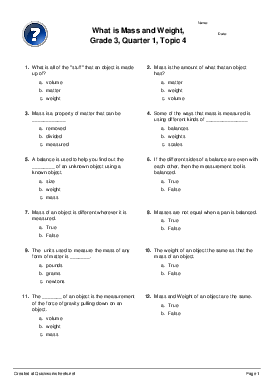# What is Mass and Weight, Grade 3, Quarter 1, Topic 4

Multiple Choice Worksheet

Kindly Shared By:United States of America

Date Shared: 12 October 2016

Worksheet Type:

Tags Describing Content or Audience:

Worksheet Instructions:

None provided.Target Language or Knowledge:

What is all of the "stuff" that an object is made up of? Mass is the amount of what that an object has? Mass is a property of matter that can be ____________ Some of the ways that mass is measured is using different kinds of _______________ A balance is used to help you find out the ________ of an unknown object using a known object. If the different sides of a balance are even with each other, then the measurement tool is balanced. Mass of an object is different wherever it is measured. Masses are not equal when a pan is balanced. The units used to measure the mass of any form of matter is _______. The weight of an object the same as that the mass of an object. The _______ of an object is the measurement of the force of gravity pulling down on an object. Mass and Weight of an object are the same. Weight of an object is different when you are in a different place. The weight of an object on the moon is the same as the weight on Earth Volume can be measured for every property type - solids, liquids and gases. _____________is the amount of space that matter occupies. On every planet, the weight of matter/object is __________ because the force of gravity. A ____________ is used to measure the weight of an object. The metric units used by scientist to measure the weight of an object is _____________. ________________ is measured by two different unit systems - Fahrenheit and Celsius. The __________rhyme says thirty is hot, twenty is nice, ten is cool and zero is ice.

matter weight volume matter weight volume measured removed divided balances scales weights mass weight size True False False True False True grams newtons pounds False True weight mass volume False True True False False True True False Volume Mass Weight different same equal spring scale pan balance triple balance newtons grams pounds temperature volume mass celsius fareneheit temperature12 October 2016

#### cabutler017AuthorUnited States of America

Last on the Mass and Weight Grade 3 worksheets